Examples

Chapter 7 Class 11 Binomial Theorem
Serial order wise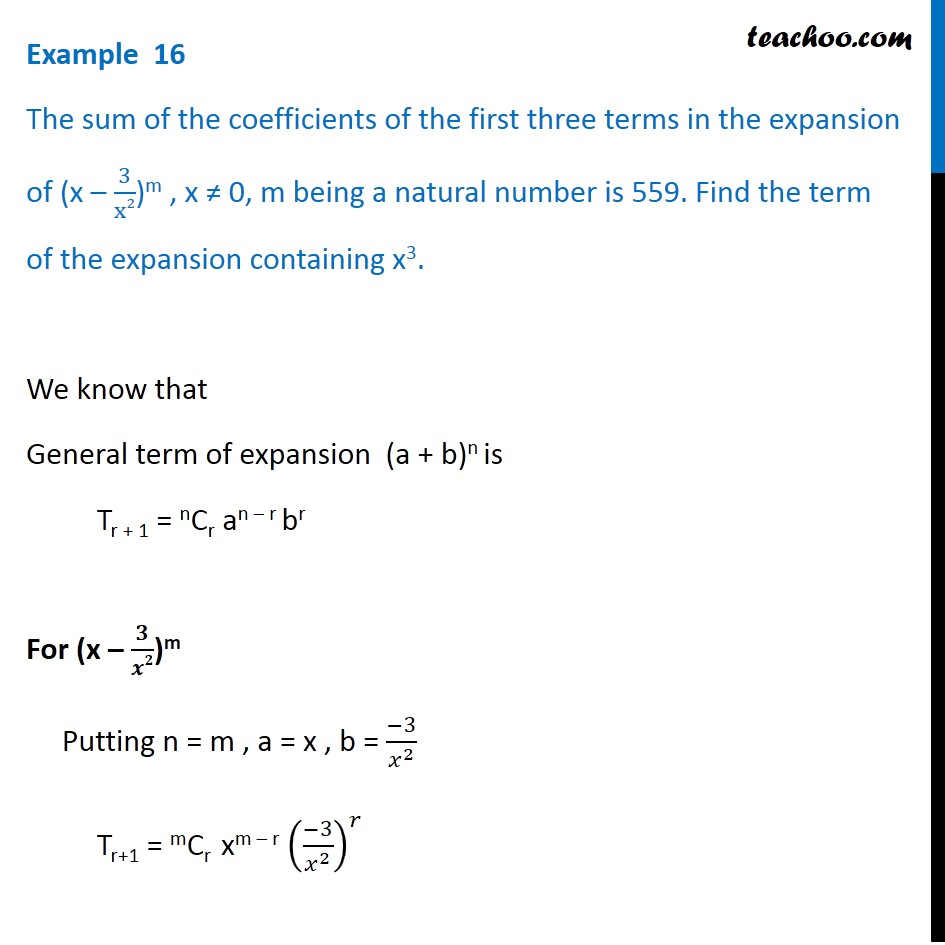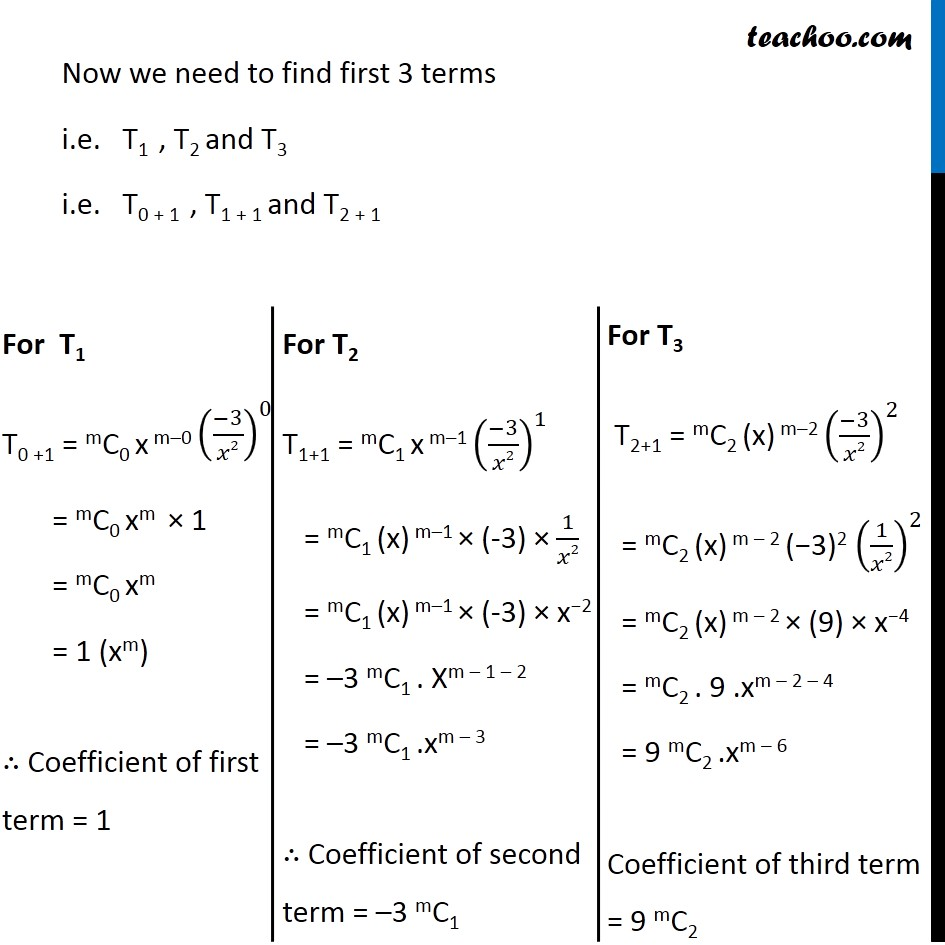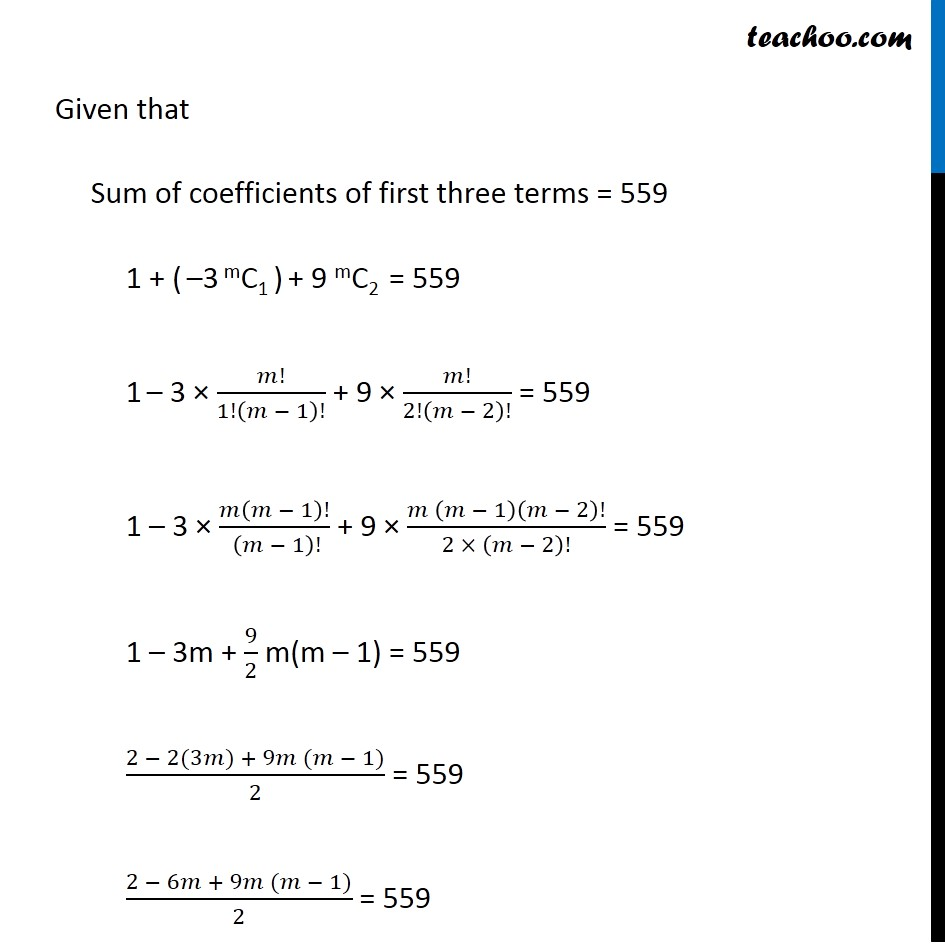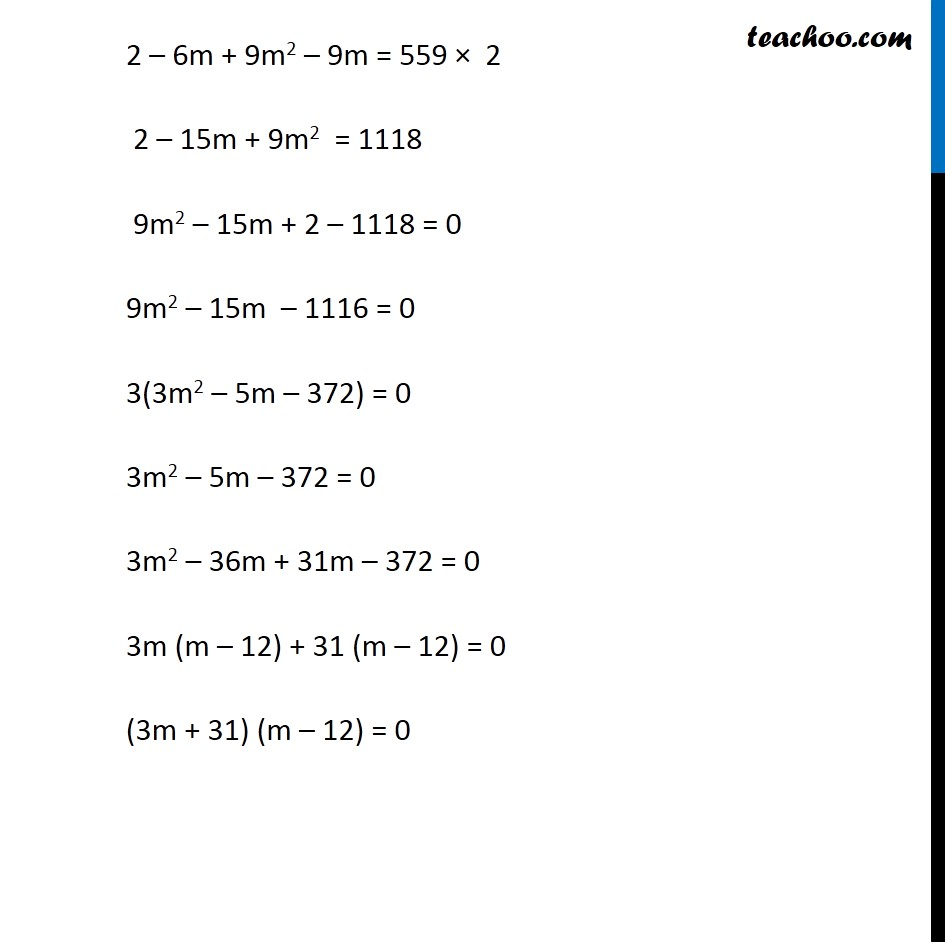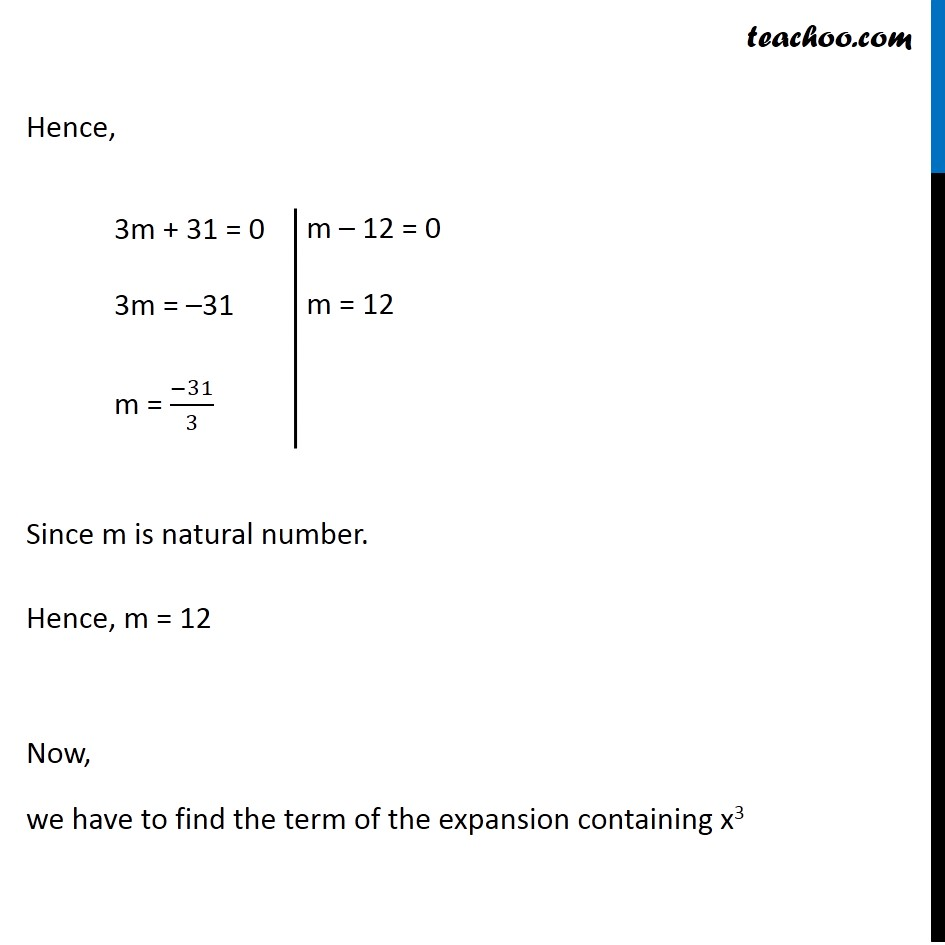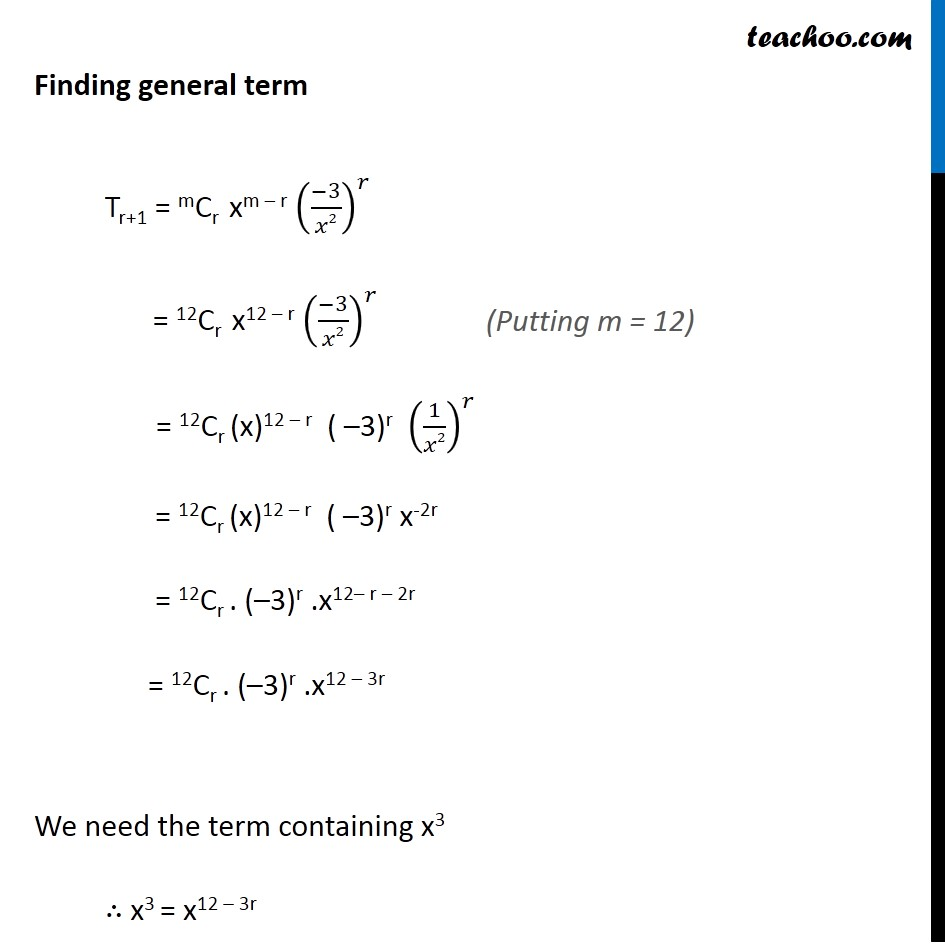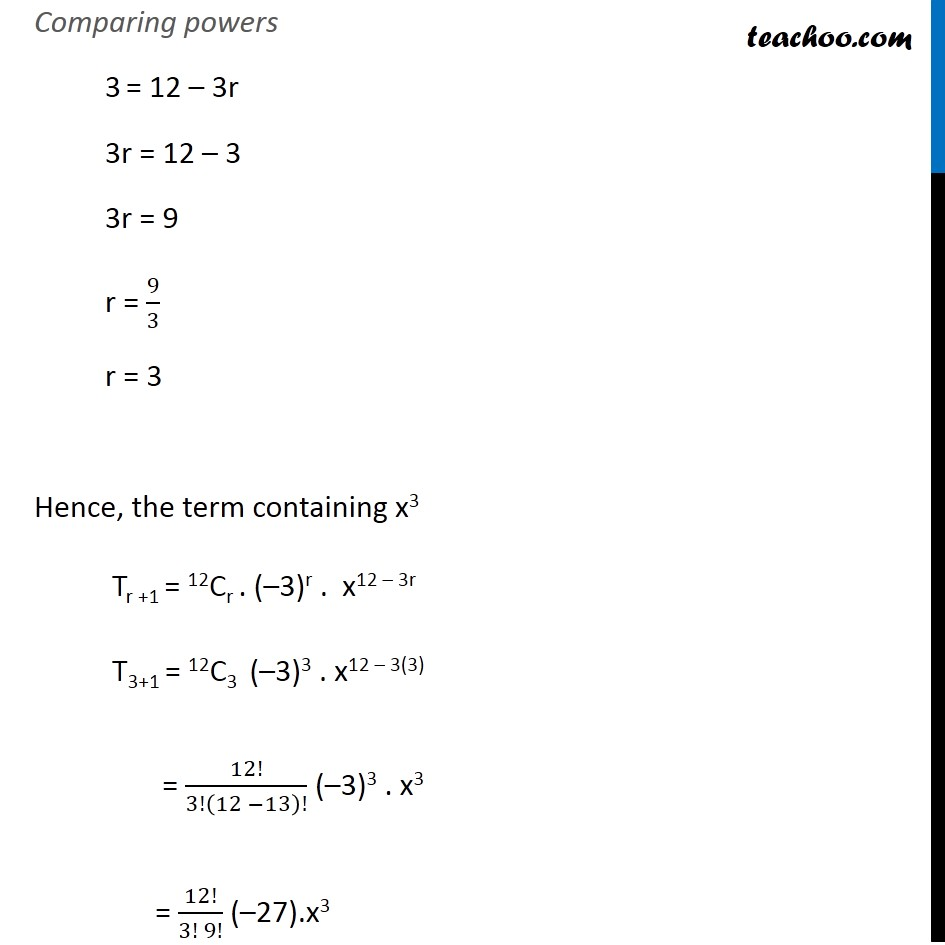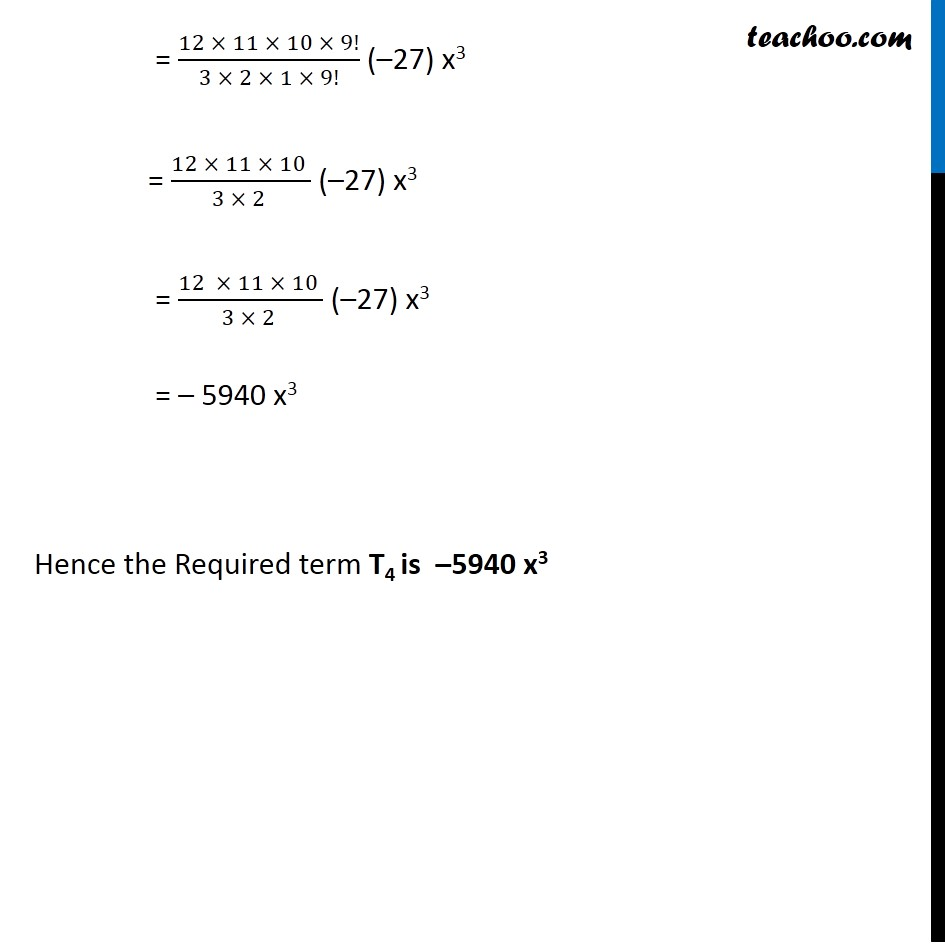Learn in your speed, with individual attention - Teachoo Maths 1-on-1 Class

### Transcript

Example 16 The sum of the coefficients of the first three terms in the expansion of (x – 3/x2)m , x ≠ 0, m being a natural number is 559. Find the term of the expansion containing x3. We know that General term of expansion (a + b)n is Tr + 1 = nCr an – r br For (x – 𝟑/𝒙𝟐)m Putting n = m , a = x , b = (−3)/𝑥^2 Tr+1 = mCr xm – r ((−3)/𝑥^2 )^𝑟 Now we need to find first 3 terms i.e. T1 , T2 and T3 i.e. T0 + 1 , T1 + 1 and T2 + 1 For T1 T0 +1 = mC0 x m–0 ((−3)/𝑥2)^0 = mC0 xm × 1 = mC0 xm = 1 (xm) ∴ Coefficient of first term = 1 For T2 T1+1 = mC1 x m–1 ((−3)/𝑥2)^1 = mC1 (x) m–1 × (-3) × 1/𝑥2 = mC1 (x) m–1 × (-3) × x−2 = –3 mC1 . Xm – 1 – 2 = –3 mC1 .xm – 3 ∴ Coefficient of second term = –3 mC1 For T3 T2+1 = mC2 (x) m–2 ((−3)/𝑥2)^2 = mC2 (x) m – 2 (−3)2 (1/𝑥2)^2 = mC2 (x) m – 2 × (9) × x−4 = mC2 . 9 .xm – 2 – 4 = 9 mC2 .xm – 6 Coefficient of third term = 9 mC2 Given that Sum of coefficients of first three terms = 559 1 + ( –3 mC1 ) + 9 mC2 = 559 1 – 3 × 𝑚!/1!(𝑚 − 1)! + 9 × 𝑚!/2!(𝑚 − 2)! = 559 1 – 3 × 𝑚(𝑚 − 1)!/( (𝑚 − 1)!) + 9 × (𝑚 (𝑚 − 1)(𝑚 − 2)!)/(2 × (𝑚 − 2)!) = 559 1 – 3m + 9/2 m(m – 1) = 559 (2 − 2(3𝑚) + 9𝑚 (𝑚 − 1))/2 = 559 (2 − 6𝑚 + 9𝑚 (𝑚 − 1))/2 = 559 2 – 6m + 9m2 – 9m = 559 × 2 2 – 15m + 9m2 = 1118 9m2 – 15m + 2 – 1118 = 0 9m2 – 15m – 1116 = 0 3(3m2 – 5m – 372) = 0 3m2 – 5m – 372 = 0 3m2 – 36m + 31m – 372 = 0 3m (m – 12) + 31 (m – 12) = 0 (3m + 31) (m – 12) = 0 Hence, Since m is natural number. Hence, m = 12 Now, we have to find the term of the expansion containing x3 3m + 31 = 0 3m = –31 m = (−31)/3 m – 12 = 0 m = 12 Finding general term Tr+1 = mCr xm – r ((−3)/𝑥2)^𝑟 = 12Cr x12 – r ((−3)/𝑥2)^𝑟 = 12Cr (x)12 – r ( –3)r (1/𝑥2)^𝑟 = 12Cr (x)12 – r ( –3)r x-2r = 12Cr . (–3)r .x12– r – 2r = 12Cr . (–3)r .x12 – 3r We need the term containing x3 ∴ x3 = x12 – 3r (Putting m = 12) Comparing powers 3 = 12 – 3r 3r = 12 – 3 3r = 9 r = 9/3 r = 3 Hence, the term containing x3 Tr +1 = 12Cr . (–3)r . x12 – 3r T3+1 = 12C3 (–3)3 . x12 – 3(3) = 12!/3!(12 −13)! (–3)3 . x3 = 12!/(3! 9!) (–27).x3 = (12 × 11 × 10 × 9!)/(3 × 2 × 1 × 9!) (–27) x3 = (12 × 11 × 10 )/(3 × 2 ) (–27) x3 = (12 × 11 × 10 )/(3 × 2 ) (–27) x3 = – 5940 x3 Hence the Required term T4 is –5940 x3